# Newtons First Law Newtons Second Law Newtons Third

• Slides: 31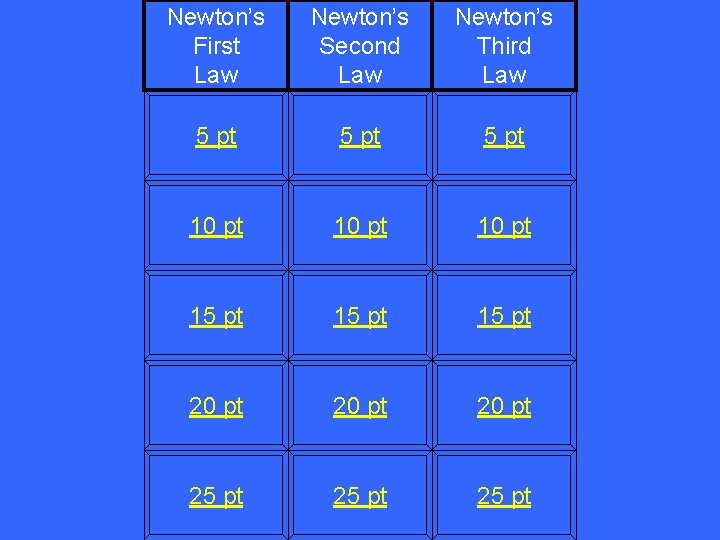Newton’s First Law Newton’s Second Law Newton’s Third Law 5 pt 10 pt 15 pt 20 pt 25 ptWhat an object at rest will do, according to Newton’s First LawWhat is stay at rest (or not move)?What an object in motion will do, according to Newton’s First LawWhat is remain in motion?The tendency of an object to resist changes in its state of motion?What is Inertia?What has to be applied to an object to change its motion, according to Newton’s First LawWhat is a force?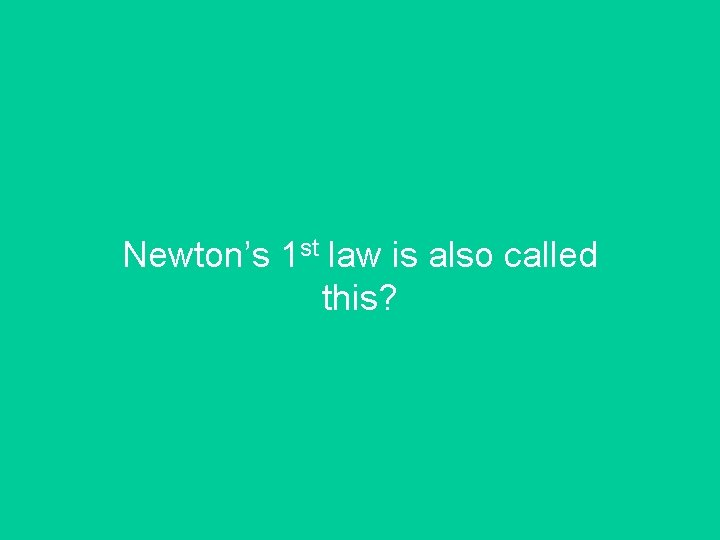Newton’s 1 st law is also called this?What is the Law of Inertia?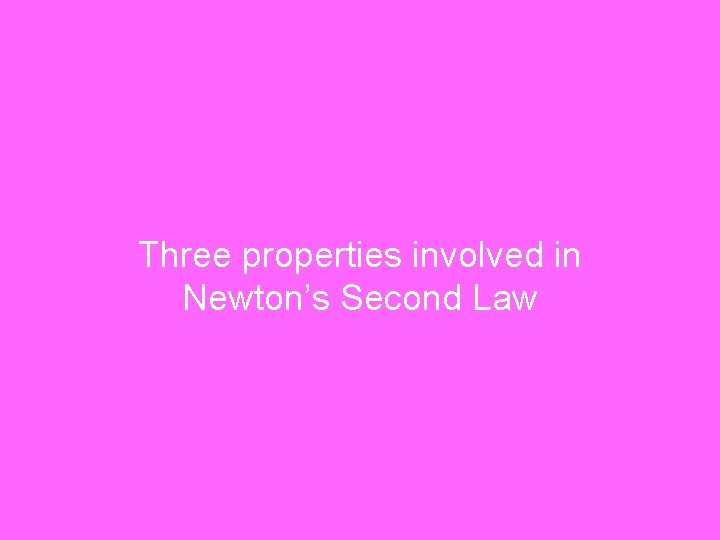Three properties involved in Newton’s Second LawWhat are force, mass and acceleration?To find the force, you would do thisWhat is multiply mass times acceleration?How to make an object accelerate twice as fastWhat is apply twice as much force (or reduce the mass by half)?To find acceleration, you would do this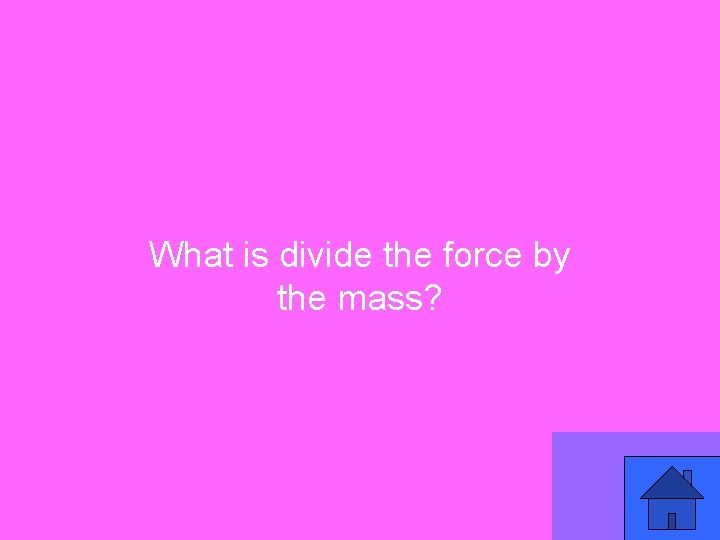What is divide the force by the mass?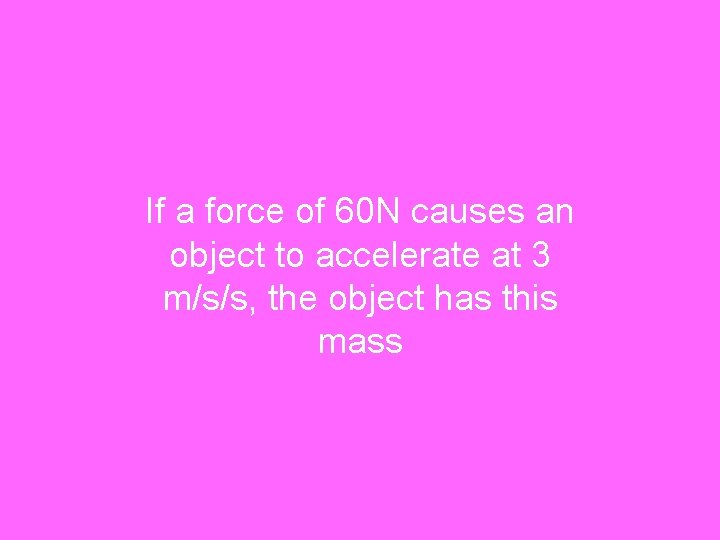If a force of 60 N causes an object to accelerate at 3 m/s/s, the object has this massWhat is 20 kg?What the wall does when you push on itWhat is pushes back?The two forces present when a ball bouncesWhat are Gravity & Friction or Action & ReactionTwo ways to describe the reaction force, according to Newton’s Third LawWhat are equal and opposite?Why your model rockets took off, according to Newton’s Third LawWhat is the force of the expelled gases from the engine ignition pushing against the launch pad?The names of the two forces involved in every pair of interactions involving Newton’s Third LawWhat are action and reaction?